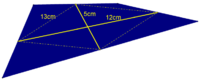# Given the dimensions shown in the diagram, find the area of the entire blue quadrilateral

#### Ypara

##### New member
When the midpoints of the adjacent sides of the blue quadrilateral are connected, they form a rhombus. Given the dimensions shown in the diagram, find the area of the entire blue quadrilateral.I didn't necessarily know how to do this problem here, but this was my best idea:
"The blue square holds 2x the area of the yellow rhombus. The area of a rhombus is 1/2 times the product of the diagonals. 2(1/2(5*12) = 60u^2"

#### brokenwatch

##### New member
I got 120cm^2 for the area of the rhombus, with the length of the diagonals being 10cm and 24cm. Half of 240 is 120. I'm don't know what this means about the quadrilateral's area, though.

#### Dr.Peterson

##### Elite Member
I presume "blue square" was a misstatement, as there is no square here. In fact, there is no rhombus in the picture (the diagonals are not shown as perpendicular), perhaps to hide something from you. So try drawing a more accurate picture (though that will not in itself make the answer obvious).

Here's one approach, which may be in part what you meant in mentioning a square: One possible quadrilateral that could generate this rhombus would be a rectangle; if the problem has an answer, the answer would have to be the area of that rectangle. What is it?

But in order to really answer the question, you need to convince yourself that the blue area is always the same. I see at least one very nice way to show that, involving triangles congruent to the four triangles outside the rhombus.